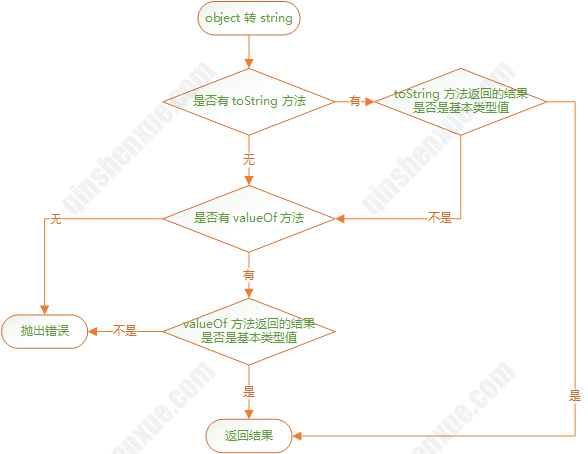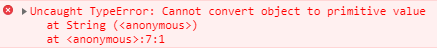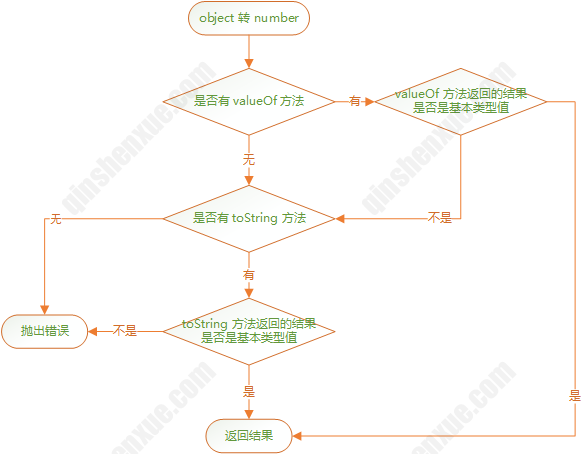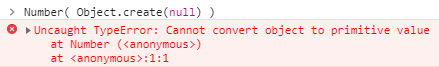# JavaScript 类型转换

2018/7/19

## 类型

JavaScript 中有 7 种类型：

nullundefinedbooleannumberstringsymbolobject

## 对象转换为基本类型值

### 1. 对象要被转换为字符串#### 举例如下

``````// 模拟 toString 返回的不是基本类型值
var obj = {
toString: function() {
return {}
}
}

String(obj)  // Uncaught TypeError: Cannot convert object to primitive value
````````````// 模拟 toString 返回的不是基本类型值，valueOf 返回的基本类型值
var obj = {
toString: function() {
return {}
},
valueOf:function(){
return null
}
}

String(obj)   // "null"
``````
``````// 模拟 toString 返回的不是基本类型值，valueOf 返回的不是基本类型值
var obj = {
toString: function() {
return {}
},
valueOf:function(){
return {}
}
}

String(obj)   // Uncaught TypeError: Cannot convert object to primitive value
``````

### 2. 对象要被转换为数字#### 举例如下

``````var obj = {
valueOf:function(){
return null
},
toString:function(){
return 1
}
}

Number(obj)  // 0  valueOf 返回的 null 转换为数字为 0
``````
``````// valueOf 和 toString 返回的都不是基本类型值
var obj = {
valueOf:function(){
return {}
},
toString:function(){
return {}
}
}

Number(obj)  // Uncaught TypeError: Cannot convert object to primitive value
``````

Object.create(null) 创建的对象没有 valueOf 和 toString 方法，因此转换时会报错。## 显式强制类型转换

### 转换为字符串

``````var obj = {
toString:function(){
return {}
}
}

String(obj) // Uncaught TypeError: Cannot convert object to primitive value

obj + ""   // Uncaught TypeError: Cannot convert object to primitive value

obj.toString()  // {}
``````

``````String( [] ) // ''
String( [null] )  // ''
String( [null, null] )  // ','
String( [undefined] ) // ''
String( [undefined, undefined] ) // ','
String( [{}] ) // '[object Object]'
String( [{toString:function(){return 1}}] ) // '1'
``````

### 转换为布尔类型

nullundefinedfalse+0-0NaN""

### 转换为数字类型

``````Number('')    // 0
Number(null)  // 0
Number(undefined)  // NaN
Number(true)  // 1
Number(false)  // 0
``````

``````Number([])  // 0

// [] valueOf 返回的是 []，因此继续调用 toString 得到基本类型值 ""，转换为数字为 0
``````

## 隐式强制类型转换

### 转换为字符串

``````var obj = {
valueOf: function() {
return 1
},
toString: function() {
return 2
}
}

obj + ''  // '1'
``````

### 转换为布尔值

1. if (..)语句中的条件判断表达式。
2. for ( .. ; .. ; .. )语句中的条件判断表达式（第二个）。
3. while (..)和do..while(..)循环中的条件判断表达式。
4. ? :中的条件判断表达式。
5. 逻辑运算符 ||（逻辑或）和 &&（逻辑与）左边的操作数（作为条件判断表达式）。

### 转换为数字类型

``````+ '2'  // 2
'2' - 0  // 2
'2' / 1   // 2
'2' * 1   // 2

+ 'x'  // NaN
'x' - 0 // NaN
'x' / 1 // NaN
'x' * 1  // NaN

1 + '2'  // '12'
1 + + '2'  // 3    即：1 + (+ '2')
``````

## == 比较

### 4、null 和 undefined

`null == undefined`， 其他类型和 null 均不相等，undefined 也是如此。

### 5、特殊情况

``````NaN == NaN  // false
-0  == +0   // true
``````

### 举例

``````"0" == false // true

// 第2条规则，false 转换为数字，结果为 0，等式变为 "0" == 0
// 两边类型不一致，继续转换，第1条规则，"0" 转换为数字，结果为 0，等式变为 0 == 0

false == [] // true

// 第3条规则，[] 转换基本类型值，[].toString()，结果为 ""，等式变为 "" == false
// 两边类型不一致，继续转换，第2条规则，false 转换为数字，结果为 0，等式变为 "" == 0
// 两边类型不一致，继续转换，第1条规则，"" 转换为数字，结果为 0，等式变为 0 == 0

0 == []    // true

// 第3条规则，[] 转换基本类型值，[].toString()，结果为 ""，等式变为 0 == ""
// 两边类型不一致，继续转换，第1条规则，"" 转换为数字，结果为 0，等式变为 0 == 0
``````

## 抽象关系比较

### 比较规则

1. 比较双方首先转换为基本类型，对象按转换为数字的流程进行转换；
2. 若有一方不是字符串，则将其转换为数字再进行比较；
3. 若有一方是 NaN，结果总是 false。

### 举例

``````null >= 0 // true
null <= 0 // true
null == 0 // flase
``````

``````var obj = {}

obj >= 0 // false
obj <= 0 // false
obj == 0 // false
``````

## 对象转换总结

``````var obj = {
valueOf:function(){
return 1
},
toString:function(){
return 2
}
}

Number(obj) // 1
String(obj) // '2'
``````

``````var obj = {
valueOf:function(){
return 1
},
toString:function(){
return 2
}
}

+obj      // 1
obj - 0   // 1
obj + ''  // '1'
obj == 1  // true
obj > 1   // false
obj < 1   // false
``````

``````new String(1).valueOf()    // '1'
new String(1).toString()   // '1'

new Number(1).valueOf()    // 1
new String(1).toString()   // '1'

new Boolean(1).valueOf()   // true
new Boolean(1).toString()  // 'true'

new Date().valueOf()       // 1532571424467
new Date().toString()      // "Thu Jul 26 2018 10:17:19 GMT+0800 (中国标准时间)"

var arr=[1,2,3]
arr.valueOf() === arr   // true
arr.toString()          // "1,2,3"

var obj = {a:1}
obj.valueOf() === obj   // true
obj.toString()          // "[object Object]"

var fun = function(){}
fun.valueOf() === fun   // true
fun.toString()          // "function(){}"
``````